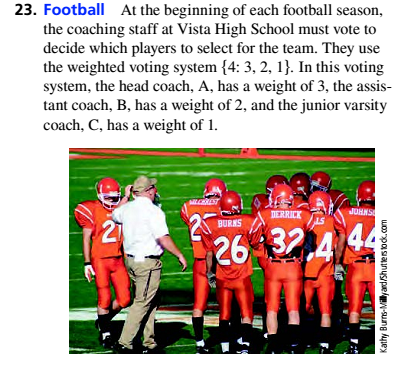# Football At the beginning of each football season, the coaching staff at Vista High School must vote to decide which players to select for the team. They use the weighted voting system {4: 3. 2. 1). In this voting system, the head coach. A. has a weight of 3, the assist ant coach. B. has a weight of 2, and the junior varsity coach. C. has a weight of I. a. Compute the Banzhaf power index for each of the coaches. b. Explaintiy it seems reasonable that the assisant coach and the junior varsity coach have the same Banzhaf power index in this voting system.### Mathematical Excursions (MindTap C...

4th Edition
Richard N. Aufmann + 3 others
Publisher: Cengage Learning
ISBN: 9781305965584

#### Solutions

Chapter
Section### Mathematical Excursions (MindTap C...

4th Edition
Richard N. Aufmann + 3 others
Publisher: Cengage Learning
ISBN: 9781305965584
Chapter 4.3, Problem 23ES
Textbook Problem
1 views

## Football At the beginning of each football season, the coaching staff at Vista High School must vote to decide which players to select for the team. They use the weighted voting system {4: 3. 2. 1). In this voting system, the head coach. A. has a weight of 3, the assist ant coach. B. has a weight of 2, and the junior varsity coach. C. has a weight of I.a. Compute the Banzhaf power index for each of the coaches.b. Explaintiy it seems reasonable that the assisant coach and the junior varsity coach have the same Banzhaf power index in this voting system.

To determine

(a)

Compute the Banzhaf power index for each of the coaches.

### Explanation of Solution

Given Information:

Consider three people the head coach, A, the assistant coach, B, and the junior varsity C, and weighted system is {4:3,2,1}.

Concept used:

BPI(v)=Number of times voter v is a critical voterNuner of times any voter is a critical voter

Calculation:

In above table we look in Critical voters column and find that A is a Critical voter three times, B is a critical voter is 1 time, and C is a critical voter is one time, therefore, Formula of Banzhaf power index of a voter v, symbolized by vis given by

BPI(v)=Number of times voter v is a critical voterNuner of times any voter is a critical voter

Then

BPI(A)=33+1+1=35=0

To determine

(b)

Explain why it seems reasonable that the assistant coach and the junior varsity coach have the same Banzhaf power index in this voting system.

### Still sussing out bartleby?

Check out a sample textbook solution.

See a sample solution

#### The Solution to Your Study Problems

Bartleby provides explanations to thousands of textbook problems written by our experts, many with advanced degrees!

Get Started

Find more solutions based on key concepts
12. Is if?

Mathematical Applications for the Management, Life, and Social Sciences

Find an equation of the line that passes through (1, 2) and (4, 5).

Applied Calculus for the Managerial, Life, and Social Sciences: A Brief Approach

Finding Derivatives In Exercises 51-62, find f(x). f(x)=4x33x2+2x+5x2

Calculus: An Applied Approach (MindTap Course List)

Finding the Magnitude In Exercises 7176, find the magnitude of v. v = 1,0,3

Calculus: Early Transcendental Functions (MindTap Course List)

What is a factorial?

Mathematics: A Practical Odyssey

Selecting midpoints of subintervals for xi to approximate the area under f(x) = 10x + 5 between x = 1 and x = 4...

Study Guide for Stewart's Single Variable Calculus: Early Transcendentals, 8th

For , f(x) = 0 2 3 f(3) does not exist

Study Guide for Stewart's Multivariable Calculus, 8th